## source of sinusoidal electromagnetic waves radiates uniformly in all directions. At a distance of 10.0 m from this source, the amplitude of

Question

source of sinusoidal electromagnetic waves radiates uniformly in all directions. At a distance of 10.0 m from this source, the amplitude of the electric field is measured to be 3.50 N>C. What is the electric-field amplitude 20.0 cm from the source

in progress 0
5 months 2021-08-05T22:11:38+00:00 1 Answers 19 views 0

## Answers ( )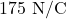Explanation: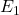= Initial electric field = 3.5 N/C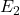= Final electric field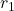= Initial distance = 10 m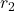= Final distance = 20 cm

Electric field is given by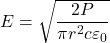So,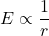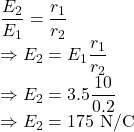The electric field amplitude at the required point is.